# Search Results (14)

View
Selected filters:
• Rates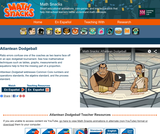Rating

Ratio errors confuse a dodgeball coach as two teams face off in an epic tournament. See how mathematical techniques such as tables, graphs, measurements and equations help to find the missing part of a proportion.

Subject:
Mathematics
Material Type:
Lecture
Provider:
Learning Games Lab
Author:
NMSU Learning Gams Lab
07/15/2015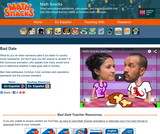Rating

True love has the right ratio. In this humorous animation, the number of words spoken by each partner predicts whether a date goes well or horribly. What do you do when someone asks if you listen to country music backwards, but won't let you get a word in edgewise?

Subject:
Mathematics
Material Type:
Lecture
Provider:
Learning Games Lab
Author:
NMSU Learning Games Lab
07/15/2015Conditions of Use:
Remix and Share
Rating

Subject:
Mathematics
Material Type:
Full Course
Provider:
Pearson
10/06/2016Conditions of Use:
Remix and Share
Rating

Putting Math to Work

Type of Unit: Problem Solving

Prior Knowledge

Students should be able to:

Solve problems with rational numbers using all four operations.
Write ratios and rates.
Use a rate table to solve problems.
Write and solve proportions.
Use multiple representations (e.g., tables, graphs, and equations) to display data.
Identify the variables in a problem situation (i.e., dependent and independent variables).
Write formulas to show the relationship between two variables, and use these formulas to solve for a problem situation.
Draw and interpret graphs that show the relationship between two variables.
Describe graphs that show proportional relationships, and use these graphs to make predictions.
Interpret word problems, and organize information.
Graph in all quadrants of the coordinate plane.

Lesson Flow

As a class, students use problem-solving steps to work through a problem about lightning. In the next lesson, they use the same problem-solving steps to solve a similar problem about lightning. The lightning problems use both rational numbers and rates. Students then choose a topic for a math project. Next, they solve two problems about gummy bears using the problem-solving steps. They then have 3 days of Gallery problems to test their problem-solving skills solo or with a partner. Encourage students to work on at least one problem individually so they can better prepare for a testing situation. The unit ends with project presentations and a short unit test.

Subject:
Mathematics
Material Type:
Unit of Study
Provider:
PearsonConditions of Use:
Remix and Share
Rating

Students work in a whole-class setting, independently, and with partners to design and implement a problem-solving plan based on the mathematical concepts of rates and multiple representations (e.g., tables, equations, and graphs). They analyze a rule of thumb and use this relationship to calculate the distance in miles from a viewer's vantage point to lightning.Key ConceptsThroughout this unit, students are encouraged to apply the mathematical concepts they have learned over the course of this year to new settings. Help students develop and refine these problem-solving skills:Creating a problem-solving plan and implementing the plan systematicallyPersevering through challenging problems to find solutionsRecalling prior knowledge and applying that knowledge to new situationsMaking connections between previous learning and real-world problemsCommunicating their approaches with precision and articulating why their strategies and solutions are reasonableCreating efficacy and confidence in solving challenging problems in the real worldGoals and Learning ObjectivesCreate and implement a problem-solving plan.Organize and interpret data presented in a problem situation.Analyze the relationship between two variables.Create a rate table to organize data and make predictions.Apply the relationship between the variables to write a mathematical formula and use the formula to solve problems.Create a graph to display proportional relationships, and use this graph to make predictions.Articulate strategies, thought processes, and approaches to solving a problem, and defend why the solution is reasonable.

Subject:
Algebra
Material Type:
Lesson Plan
Provider:
PearsonConditions of Use:
Remix and Share
Rating

Four full-year digital course, built from the ground up and fully-aligned to the Common Core State Standards, for 7th grade Mathematics. Created using research-based approaches to teaching and learning, the Open Access Common Core Course for Mathematics is designed with student-centered learning in mind, including activities for students to develop valuable 21st century skills and academic mindset.

Subject:
Mathematics
Material Type:
Full Course
Provider:
Pearson
10/06/2016Conditions of Use:
Remix and Share
Rating

Getting Started

Type of Unit: Introduction

Prior Knowledge

Students should be able to:

Understand ratio concepts and use ratios.
Use ratio and rate reasoning to solve real-world problems.
Identify and use the multiplication property of equality.

Lesson Flow

This unit introduces students to the routines that build a successful classroom math community, and it introduces the basic features of the digital course that students will use throughout the year.

An introductory card sort activity matches students with their partner for the week. Then over the course of the week, students learn about the routines of Opening, Work Time, Ways of Thinking, Apply the Learning (some lessons), Summary of the Math, Reflection, and Exercises. Students learn how to present their work to the class, the importance of students’ taking responsibility for their own learning, and how to effectively participate in the classroom math community.

Students then work on Gallery problems, to further explore the resources and tools and to learn how to organize their work.

The mathematical work of the unit focuses on ratios and rates, including card sort activities in which students identify equivalent ratios and match different representations of an equivalent ratio. Students use the multiplication property of equality to justify solutions to real-world ratio problems.

Subject:
Mathematics
Material Type:
Unit of Study
Provider:
PearsonConditions of Use:
Remix and Share
Rating

Review the ways classroom habits and routines can strengthen students’ mathematical character. Explain what a Gallery is and how to choose a Gallery problem to solve. Direct students to choose one of three Gallery problems that introduce the unit’s technology resources. The three Gallery problems combine working with ratios and rates with the application resources available with this unit.Key ConceptsStudents understand that a Gallery gives them a choice of problems to solve. Students think about the features of the problems to use when choosing a problem. Students know how to work on a Gallery problem and present a solution.Goals and Learning ObjectivesKnow how to choose a problem from a Gallery.

Subject:
Ratios and Proportions
Material Type:
Lesson Plan
Provider:
Pearson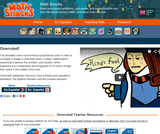Rating

Two besotted rulers must embrace proportional units in order to unite their lands. It takes mathematical reasoning to identify the problem, and solution, when engineers from Queentopia and Kingopolis build a bridge to meet in the middle of the river.

Subject:
Mathematics
Material Type:
Lecture
Provider:
Learning Games Lab
Author:
NMSU Learning Games Lab
07/15/2015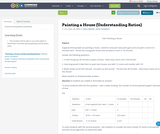Rating

A task involving Ratios and Rates

Subject:
Mathematics
Material Type:
Activity/Lab
Author:
Elana Webster
James Strickland
01/28/2016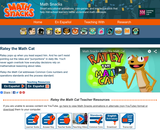Rating

Ratey pops up when you least expect him. And he can't resist pointing out the rates and "purrportions" in daily life. It turns out that everyday decisions rely on mathematical reasoning about rates.

Subject:
Mathematics
Material Type:
Diagram/Illustration
Lecture
Provider:
Learning Games Lab
Author:
NMSU Learning Games Lab
07/15/2015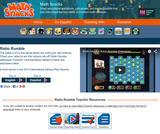Rating

The battle is on in this game where you build your own potions! Check your ratios to win this mixture mix-off. Ratio Rumble guides students in: identifying ratios when used in a variety of contextual situations; providing visual representations of ratios; solving common problems or communicating by using rate, particularly unit rates; and explaining why ratios and rates naturally relate to fractions and decimals.

Subject:
Mathematics
Material Type:
Game
Interactive
Provider:
Learning Games Lab
Author:
NMSU Learning Games Lab
07/20/2015Rating

The evil Scaleo has escaped from prison and is transforming the length, width, and height of objects until they become useless – or dangerous. Who can put things right? Superheroine Scale Ella uses the power of scale factor to foil the villain.

Subject:
Mathematics
Material Type:
Lecture
Provider:
Learning Games Lab
Author:
NMSU Learning Games Lab
07/15/2015
Remix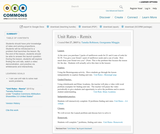Conditions of Use:
Remix and Share
Rating

Students should have prior knowledge of rates and solving proportions. Students will be introduced to a problem that launches the lesson. By the end of the lesson, students should be able to answer the launch question. During the lesson, students will explore finding the unit rate, watch a video demonstration, and practice via whiteboards and interactively.

Subject:
Mathematics
Material Type:
Lesson Plan
Author:
Tameka Robinson
Georgeanna Minggia